## Percent to Fraction Calculator

##### Калькулятор онлайн преобразование процентов
%

The calculator will convert percent into fraction (proper, improper, mixed number), with steps shown. f the percent value is greater than 100% it is converted into a mixed number fraction.

##### How to Convert a Percent to a Fraction - Steps

To convert a Percent to a Fraction follow these steps:

• 1 Write down the percent divided by 100 like this: 100:• 2 If the percent is not a whole number, then multiply both top and bottom by 10 for every number after the decimal point.
• 3 Simplify (or reduce) the fraction.
##### Example Convert the percent 22.25% into fraction.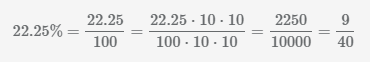.

Simplify (or reduce) the above fraction by dividing both numerator and denominator by the GCD(2250,10000)=250.

##### Example Convert the percent 40% into fraction.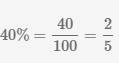.

Simplify (or reduce)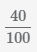the above fraction by dividing both numerator and denominator by the GCD(40,100)=20.

##### Example Convert 1% to a fraction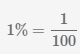. One percent is equal to one hundredth.

##### Example Convert the percent 17.125% into fraction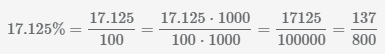.

##### Example Convert the percent 50.75% into fraction.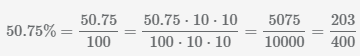.